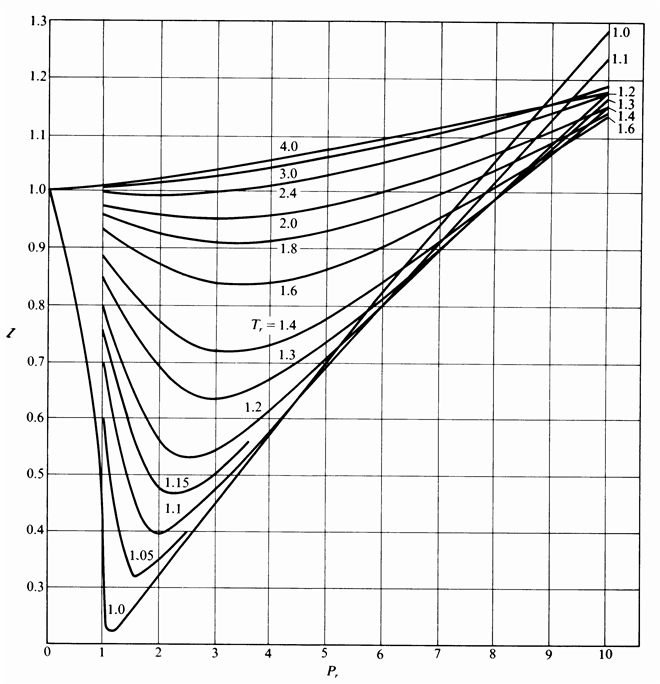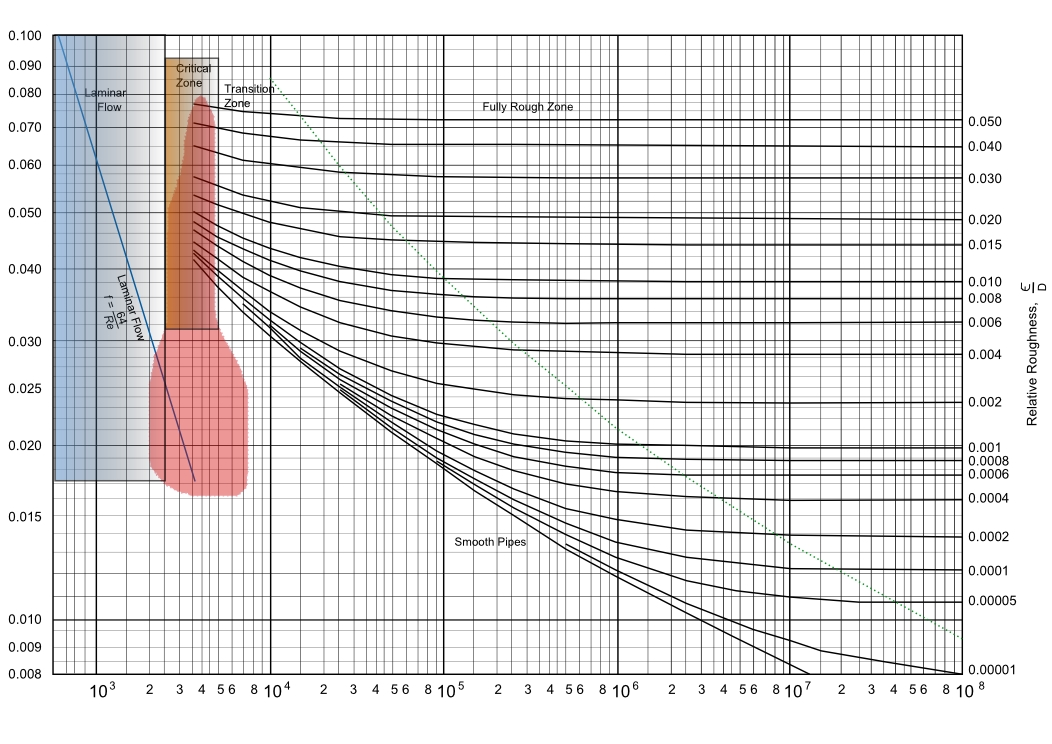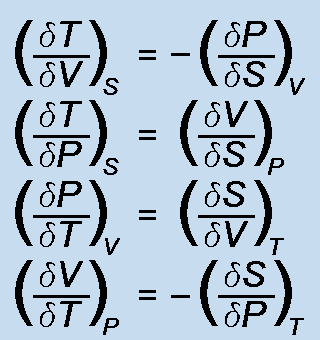### ChemE Soft StoreCaCO3 -> CaO + CO2
NaCl + NH3 + CO2 + H2O -> NaHCO3 + NH4Cl
2 NaHCO3 -> Na2 CO3 + H2O + CO2
CaO + H2 O -> Ca(OH)2
Ca(OH)2 + 2 NH4 Cl -> CaCl2 + 2 NH3 + 2 H2O

Conversions

Convert quantities between different units. Conversions between common English units and metric, includes temperature scale conversions, densities, viscosities, energy, power, force, heat transfer co-efficients, etc.

Vector Math

Vector math includes three dimensional conversion between cartesian, cylindrical, and spherical co-ordinate systems. Also addition, angle of intersection, dot and cross product solutions.

Root Solver

Interpolation/extrapolation. Solve for the roots of quadratic, cubic, and quartic equations, as well as finding the solutions to functions of x -> f(x). Includes complex number math solutions as well.

Matrix Math

Solve linear equations up to 6x6 matrices. Results show the solution and inverse matrices, the determinant, as well as the matrices of determinants and minors.

2 Parm Regression

Two parameter regression analysis solving for eight different functions. Functions are plotted as well as correlation coefficients calculated. Functions include the temperature dependent Arrhenius equation.

Poly Regression

Polynomial regression analysis of x-y data for six orders of the polynomial equation. Specified orders are solved, plotted, and correlation coefficients calculated.

Multi Regression

Regression analysis of multivariable data up to four independent variables. Each independent variable is solved, plotted, and correlation coefficients calculated.

### Error Function

Error function calculations for thermal transport in solids. Temperature can be calculated for position and time with boundary conditions set for the limits.